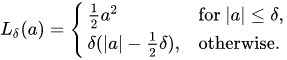# Defining a Custom Loss Function - Huber Loss

Let's implement huber loss. Huber loss is less sensitive to outliers in data than mean squared error.

Below is the formula of huber loss.Note:

• Huber loss is defined as:

• error 2/2, if error < delta (ie, if it is a small error)

• delta * ( |error| - delta/2), otherwise ( |error| means the absolute value error)

In this exercise, we consider delta=1.

Thus, the `huber_fn` is defined as:

• error 2/2, if error < 1 (ie, if it is a small error).

• |error| - 0.5, otherwise

• `tf.abs(x)` returns the positive value(absolute value) of `x`.

• `tf.square(x)` returns the squared value of `x`.

• `tf.where(bool_array, x, y)` returns the elements where condition is True in `bool_array` (multiplexing `x` and `y`).

In simpler terms, `tf.where` will choose an output shape from the shapes of condition, `x`, and `y` that all three shapes are broadcastable to.

The condition tensor acts as a mask that chooses whether the corresponding element/row in the output should be taken from x (if the element in the condition is `True`) or from `y` (if it is `False`).

For example, upon executing the following,

`tf.where([True, False, False, True], [1,2,3,4], [100,200,300,400])`

the output would be : `<tf.Tensor: shape=(4,), dtype=int32, numpy=array([ 1, 200, 300, 4], dtype=int32)>`

INSTRUCTIONS
• Define the `huber_fn`, the Huber Loss function, and pass the `y_true, y_pred` as input arguments to the function. We do this as follows:

• Calculate `error` which is `y_true - y_pred`

• If `tf.abs(error) < 1`, then `is_small_error` is `True`. Else, `is_small_error` is `False`.

• Define `squared_loss` as `tf.square(error) / 2`.

• Define `linear_loss` as `tf.abs(error) - 0.5`.

• Use `tf.where` and pass `is_small_error, squared_loss, linear_loss` as input arguments to it, to choose either the `squared_loss` value or the `linear_loss` value based on if the `is_small_error` condition is `True` or `False`.

• Thus, return the huber loss for each prediction.

So use the following code to do the same:

``````    def huber_fn(y_true, y_pred):
error = y_true - y_pred
is_small_error = tf.abs(error) < 1
squared_loss = tf.square(error) / 2
linear_loss  = tf.abs(error) - 0.5
return tf.where(is_small_error, squared_loss, linear_loss)
``````

No hints are availble for this assesment

Answer is not availble for this assesment

Note - Having trouble with the assessment engine? Follow the steps listed here